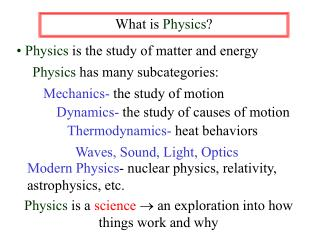# What is Physics ? - PowerPoint PPT PresentationDownload PresentationWhat is Physics ?

Download Presentation## What is Physics ?

- - - - - - - - - - - - - - - - - - - - - - - - - - - E N D - - - - - - - - - - - - - - - - - - - - - - - - - - -
##### Presentation Transcript

1. What is Physics? • Physics is the study of matter and energy Physics has many subcategories: Mechanics- the study of motion Dynamics- the study of causes of motion Thermodynamics- heat behaviors Waves, Sound, Light, Optics Modern Physics- nuclear physics, relativity, astrophysics, etc. Physics is a science an exploration into how things work and why

2. Making connections in science-- modeling and the scientific method A model is an analogy or comparison to a familiar idea, e.g. comparing light waves to water waves The Scientific Method is a problem solving procedure: Observation of a natural phenomena Hypothesis-- an educated guess Experimentation leads to Theory Wider experimentation leads to dismissal or acceptance as Law - descriptive, not restrictive

3. Physics--> the study of matter and energy Energy-- the ability to do work Which is anything! Matter: Described by its specific properties Pure Energy:Sound, Light, Heat

4. Potential Energy -gravitational -elastic -chemical -etc. Kinetic Energy the energy contained by any object that is in “motion” unless otherwise specified, the surface of the earth is considered stationary Energy-the capacity to do work

5. (Some) Properties of Matter Mass: How much stuff - quantity of matter Inertia: A resistance to a change in motion Volume: How much space the matter takes up Mass Density: the ratio of mass to volume m V D = units: g/cm3

6. This is one physical concept represented mathematically, but it is three equations: m V D = m D m = DV V = For our purposes, these are all the same base relationship for use in problem solving.

7. Problem Solving Procedure • Read through the entire problem! • List the given information (quantity with number and units). • Find and write a base equation for the given information. • Put the numbers in and solve. • Circle your final answer with correct units!

8. What is the density of a rectangular object that has a mass of 63.2 g and is 3.42 cm long, 1.09 cm wide and 2.56 cm in height? D = ? D = m V V = l• w • h m = 63.2 g l = 3.42 cm w = 1.09 cm D = m l• w • h h = 2.56 cm = 63.2 g (3.42 cm)(1.09 cm)(2.56 cm) = 6.62 g/cm3

9. The mass of a block of wood is measured to be 125.25 g. The length of the block is measured as 5.25 cm, the width is measured as 6.80 cm and the height is measured as 8.35 cm. Find the mass density of this wood block. .420 g/cm3 The density of a sample of lead is found to be 11.6 g/cm3. If the mass of the lead is measured to be 125.0 g, what must be the volume of the sample? A block of wood with dimensions of 5.35 cm, 3.45 cm and 2.45 cm has a mass density of .876 g/cm3. What must the mass of that block be? 39.6 g

10. A wooden block of mass 115.62 g has a density of .687 g/cm3. What must the height of the block be if the length is 12.35 cm and the width is 8.25 cm? A block of unknown material is found to have a mass of 245 g and dimensions of 22.0 cm, 15.0 cm, and 12.0 cm. Will this block float in water? Oh yes! What is the mass of a block of wood that has a density of .760 g/cm3 and measures 15.0 cm by 14.0 cm by 8.35 cm?

11. Explain how someone may have used the scientific method in order to solve a problem. Explain by outlining and giving examples following each step of the method. This example can be a scientist, such as Copernicus, or simply an example from every day life.